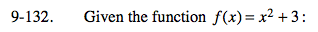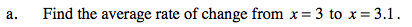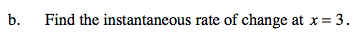### Home > PC > Chapter 9 > Lesson 9.3.3 > Problem9-132

9-132.
1. Given the function f(x) = x2 + 3 : Homework Help ✎

1. Find the average rate of change from x = 3 to x = 3.1.

2. Find the instantaneous rate of change at x = 3.$\frac{f(3.1)-f(3)}{3.1-3}$$\lim_{h\rightarrow 0}\frac{f(3+h)-f(3)}{(3+h)-3}$